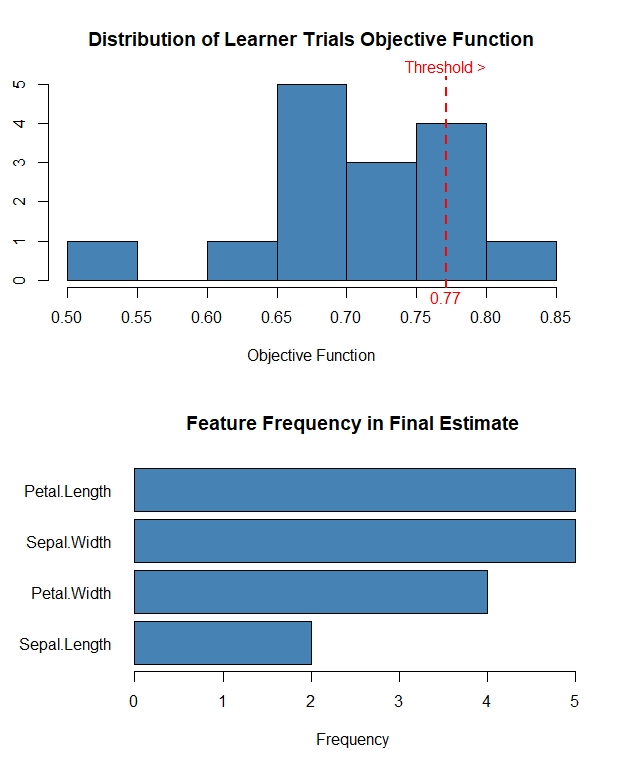# Getting Started with NNS: Classification

library(NNS)
library(data.table)
require(knitr)
require(rgl)
require(meboot)
require(plyr)
require(tdigest)
require(dtw)

# Classification

NNS.reg is a very robust regression technique capable of nonlinear regressions of continuous variables and classification tasks in machine learning problems.

We have extended the NNS.reg applications per the use of an ensemble method of classification in NNS.boost.

One major advantage NNS.boost has over tree based methods is the ability to seamlessly extrapolate beyond the current range of observations.

## Splits vs. Partitions

Popular boosting algorithms take a series of weak learning decision tree models, and aggregate their outputs. NNS is also a decision tree of sorts, by partitioning each regressor with respect to the dependent variable. We can directly control the number of “splits” with the NNS.reg(..., order = , ...) parameter.

### NNS Partitions

We can see how NNS partitions each regressor by calling the $rhs.partitions output. You will notice that each partition is not an equal interval, nor of equal length, which differentiates NNS from other bandwidth or tree-based techniques. Higher dependence between a regressor and the dependent variable will allow for a larger number of partitions. This is determined internally with the NNS.dep measure. NNS.reg(iris[,1:4], iris[,5], residual.plot = FALSE, ncores = 1)$rhs.partitions
##    Sepal.Length Sepal.Width Petal.Length Petal.Width
## 1:          4.3         2.0     1.000000         0.1
## 2:          5.0         3.0     1.398673         1.0
## 3:          6.0         4.4     4.326619         2.0
## 4:          7.9          NA     5.095300         2.5
## 5:           NA          NA     5.626123          NA
## 6:           NA          NA     6.900000          NA

# NNS.boost

Through resampling of the training set and letting each iterated set of data speak for themselves (while paying extra attention to the residuals throughout), we can test various regressor combinations in these dynamic decision trees…only keeping those combinations that add predictive value. From there we simply aggregate the predictions.

NNS.boost will automatically search for an accuracy threshold from the training set, reporting iterations remaining and level obtained in the console. A plot of the frequency of the learning accuracy on the training set is also provided.

Once a threshold is obtained, NNS.boost will test various feature combinations against different splits of the training set and report back the frequency of each regressor used in the final estimate.

Let’s have a look and see how it works. We use 140 random iris observations as our training set with the 10 holdout observations as our test set.

set.seed(1234)
test.set = sample(150,10)

a = NNS.boost(IVs.train = iris[-test.set, 1:4],
DV.train = iris[-test.set, 5],
IVs.test = iris[test.set, 1:4],
epochs = 100, learner.trials = 100,
status = FALSE,
type = "CLASS")a$results ##  1 2 3 3 3 3 3 3 2 3 a$feature.weights
## Sepal.Length  Sepal.Width Petal.Length  Petal.Width
##       0.1250       0.3125       0.3125       0.2500
mean( a$results == as.numeric(iris[test.set, 5]) ) ##  1 A perfect classification. # Cross-Validation Classification Using NNS.stack The NNS.stack() routine cross-validates for a given objective function the n.best parameter in the multivariate NNS.reg function as well as the threshold parameter in the dimension reduction NNS.reg version. NNS.stack can be used for classification via NNS.stack(..., type = "CLASS", ...). Using the reduced features (if any and calling them from names(a$feature.weights)) from our NNS.boost output, we can further cross-validate the classification using NNS.stack.

b = NNS.stack(IVs.train = iris[-test.set, names(a$feature.weights)], DV.train = iris[-test.set, 5], IVs.test = iris[test.set, names(a$feature.weights)],
type = "CLASS", stack = FALSE,
ncores = 1)

b
## $OBJfn.reg ##  0.9550416 ## ##$NNS.reg.n.best
##  2
##
## $probability.threshold ##  0.27 ## ##$OBJfn.dim.red
##  1
##
## $NNS.dim.red.threshold ##  0.77 ## ##$reg
##   1 2 3 3 3 3 3 3 2 3
##
## $dim.red ##  1 2 3 3 3 3 3 3 2 3 ## ##$stack
##   1 2 3 3 3 3 3 3 2 3
mean( b\$stack == as.numeric(iris[test.set, 5]) )
##  1

## Brief Notes on Other Parameters

• representative.sample uses a representation of each of the regressors via Tukey’s five number summary as well as mean and mode. This encoding of the regressors greatly reduces large datasets runtimes.

• depth = "max" will force all observations to be their own partition, forcing a perfect fit of the multivariate regression. In essence, this is the basis for a kNN nearest neighbor type of classification. For mixed data type regressors / features, it is suggested to use NNS.boost(..., depth = "max", ...).

• n.best = 1 will use the single nearest neighbor. When coupled with depth = "max", NNS will emulate a kNN = 1 but as the dimensions increase the results diverge demonstrating NNS is less sensitive to the curse of dimensionality than kNN.

• extreme will use the maximum threshold obtained, and may result in errors if that threshold cannot be eclipsed by subsequent iterations.